Courses

# Test: Boiler & Steam Turbine - 2

## 30 Questions MCQ Test RRB JE for Mechanical Engineering | Test: Boiler & Steam Turbine - 2

Description
This mock test of Test: Boiler & Steam Turbine - 2 for Mechanical Engineering helps you for every Mechanical Engineering entrance exam. This contains 30 Multiple Choice Questions for Mechanical Engineering Test: Boiler & Steam Turbine - 2 (mcq) to study with solutions a complete question bank. The solved questions answers in this Test: Boiler & Steam Turbine - 2 quiz give you a good mix of easy questions and tough questions. Mechanical Engineering students definitely take this Test: Boiler & Steam Turbine - 2 exercise for a better result in the exam. You can find other Test: Boiler & Steam Turbine - 2 extra questions, long questions & short questions for Mechanical Engineering on EduRev as well by searching above.
QUESTION: 1

### The equivalent evaporation (kg/hr) of a boiler producing 2000 kg/hr ofsteam with enthalpy content of 2426 kJ/kg from feed water at temperature40°C (liquid enthalpy = 168 kJ/kg, enthalpy of vaporisation of water at100°C = 2258 kJ/kg) is

Solution: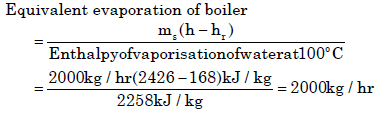QUESTION: 2

### Heat is mainly transferred by conduction, convection and radiation in

Solution:

All modes of heat transfer occur in boiler furnace

QUESTION: 3

### The shell diameter and length of locomotive boiler are

Solution:
QUESTION: 4

Match List I (Boilers) with List II (Type/Description) and select the correct answer using the codes given below the Lists:
List I                                                                     List II
(Boilers)                                                       (Type/Description)
A. Lancashire                                      1. Horizontal straight tube, fire-tube boiler
B. Benson                                           2. Inclined straight tube, water-tube boiler
C. Babcock and Wilcox                       3. Bent tube, water-tube boiler
D. Stirling                                             4. High pressure boiler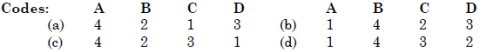Solution:
QUESTION: 5

Which one of the following statements is not true for a supercritical steam generator?

Solution:

It has no drum.

QUESTION: 6

An attemparator is used in some utility boilers

Solution:
QUESTION: 7

Assertion (A): An 'air-to-close’ valve should be used to control the fuel supply to the furnace. Reason (R): In the event of air failure, the valve would be closed and the fuels cut off to prevent overheating

Solution:
QUESTION: 8

The distinguishing feature of Velox boiler is that it

Solution:

Due to supersonic flue gas velocity in the Velox Boiler larger heat transfer from a smaller surface area is possible.

QUESTION: 9

For a single stage impulse turbine with a rotor diameter of 2 m and aspeed of 3000 rpm when the nozzle angle is 20o, the optimum velocity ofsteam in m/s is

Solution: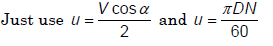QUESTION: 10

Match the following
P. Curtis                                 1. Reaction steam turbine
Q. Rateau                              2. Gas turbine
R. Kaplan                               3. Velocity compounding
S. Francis                              4. Pressure compounding
5. Impulse water turbine
6. Axial turbine
7. Mixed flow turbine
8. Centrifugal pump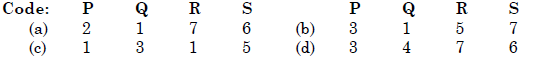Solution:
QUESTION: 11

In the velocity diagram shownbelow, u = blade velocity, C = absolute fluid velocity and ω  = relative velocity of fluid and thesubscripts 1 and 2 refer to inletand outlet.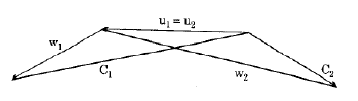Solution:
QUESTION: 12

Ambient air dry- bulb temperature is 45°C and wet bulb temperature is27°C. Select the lowest possible condensing temperature from the following for an evaporative cooled condenser.

Solution:

It should be greater than 27oC

QUESTION: 13

In steam turbine terminology, diaphragm refers to

Solution:

Diaphragm in steam turbines is a separating wall between rotors carrying nozzles.

QUESTION: 14

Which one of the following sketches represents an impulse turbine blade?

Solution:

Figure at (a) is for impulse turbine blade. As no pressure change from inlet to outlet no area change is allowed.

QUESTION: 15

The given figure shows the variation of certain steam parameter in case of a simple impulse turbine. The curve A-BC represents the variation of

Solution:
QUESTION: 16

Consider the following statements in respect of impulse steam turbines:

1. Blade passages are of constant cross-sectional area

2. Partial admission of steam is permissible.

3. Axial thrust is only due to change in flow velocity of steam at inlet and outlet of moving blade.

Which of the statements given above are correct?.

Solution:
QUESTION: 17

A single-stage impulse turbine with a diameter of 120 cm runs at 3000 rpm.If the blade speed ratio is 0.42 then, the inlet velocity of steam will be

Solution: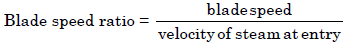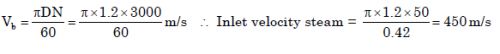QUESTION: 18

Given, Vb = Blade speed  V = Absolute velocity of steam entering the blade, α = Nozzle angle. The efficiency of an impulse turbine is maximum when

Solution:
QUESTION: 19

Which one of the following is used to bring down the speed of an impulsesteam turbine to practical limits?

Solution:
QUESTION: 20

Why is compounding of steam turbines done?

Solution:
QUESTION: 21

List I gives the various velocities in the velocity diagrams of a two-stage impulse turbine. List II gives the blade angles. Match the velocity from List I with the angle in List II and select the correct answer using the codes given below the lists: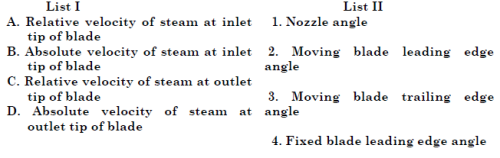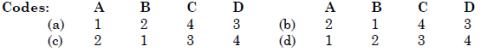Solution:
QUESTION: 22

Assertion (A): Impulse staging is commonly employed in high pressure part and reaction staging in intermediate low pressure parts of the steam turbine Reason (R): The tip leakage across moving blades is less in impulse staging as the pressure drop is small and there can be large pressure drop across fixed blades and nozzles.

Solution:
QUESTION: 23

The compounding of steam turbines is done to

Solution:

The compounding of steam turbine is done to reduce turbine speed.

QUESTION: 24

Running speeds of steam turbine can be brought down to practical limitsby which of the following method(s)?

1. By using heavy flywheel.

2. By using a quick response governor.

3. By compounding

4. By reducing fuel feed to the furnace.

Solution:
QUESTION: 25

Which one of the following is the feature of pressure compounding (Rateaustaging)?

Solution:
QUESTION: 26

Which of the following statements are correct?

1. Impulse turbine rotor blades are thick at the centre.

2. Rateau turbine is more efficient than Curtis turbine

.3. Blade velocity coefficient for an impulse turbine is of the order of 60%.

Solution:

3 is wrong because blade velocity coefficient for an impulse turbine is of the order less than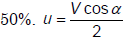QUESTION: 27

Assertion (A): Modern turbines have velocity compounding at the initial stagesand pressure compounding in subsequent stages.

Reason (R): Excessive tip leakage occurs in the high pressure region of reactionblading.

Solution:
QUESTION: 28

The net result of pressure-velocity compounding of steam turbine is:

Solution:

Pressure-velocity compounding of steam turbines results is less number of stages

QUESTION: 29

A 4-row velocity compounded steam turbine develops total 6400 kW. Whatis the power developed by the last row?

Solution:

work done in different stages in velocity compounding is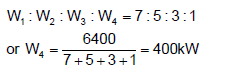QUESTION: 30

In Parson's reaction turbines, the velocity diagram triangles at the inletand outlet are which of the following?

Solution: